#Qualitative Techniques: Slope FieldsA differentiable function--and the solutions to differential equations better be differentiable--has tangent lines at every point. Let's draw small pieces of some of these tangent lines of the function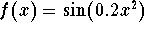: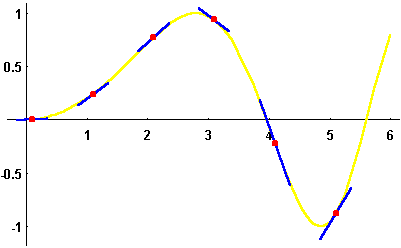Slope fields (also called vector fields or direction fields) are a tool to graphically obtain the solutions to a first order differential equation. Consider the following example: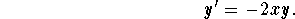The slope, y'(x), of the solutions y(x), is determined once we know the values for x and y , e.g., if x=1 and y=-1, then the slope of the solution y(x) passing through the point (1,-1) will be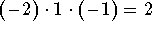. If we graph y(x) in the x-y plane, it will have slope 2, given x=1 and y=-1. We indicate this graphically by inserting a small line segment at the point (1,-1) of slope 2.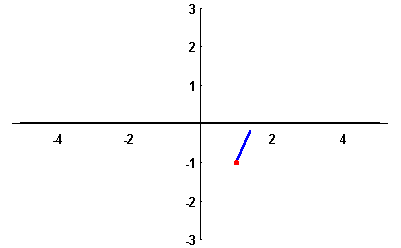Thus, the solution of the differential equation with the initial condition y(1)=-1 will look similar to this line segment as long as we stay close to x=-1.

Of course, doing this at just one point does not give much information about the solutions. We want to do this simultaneously at many points in the x-y plane.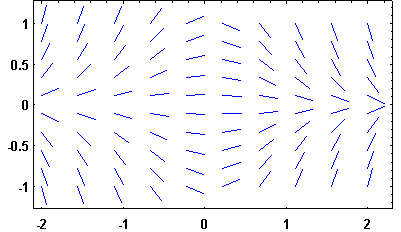We can get an idea as to the form of the differential equation's solutions by " connecting the dots." So far, we have graphed little pieces of the tangent lines of our solutions. The " true" solutions should not differ very much from those tangent line pieces!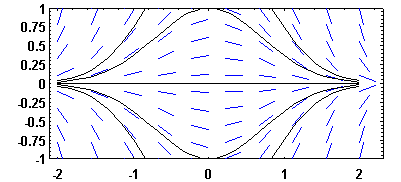Let's consider the following differential equation: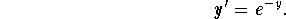Here, the right-hand side of the differential equation depends only on the dependent variable y, not on the independent variable x. Such a differential equation is called autonomous. Autonomous differential equations are always separable.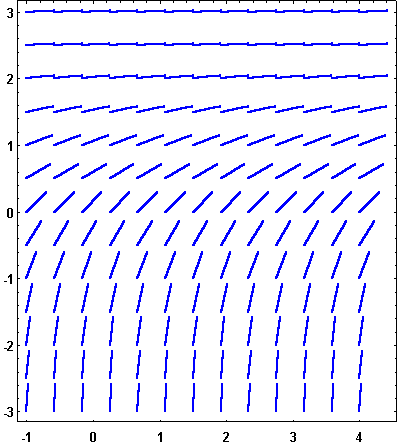Autonomous differential equations have a very special property; their slope fields are horizontal-shift-invariant, i.e. along a horizontal line the slope does not vary.#### Try it yourself!

What is special about the solutions to an autonomous differential equation?Here is an example of the logistic equation which describes growth with a natural population ceiling: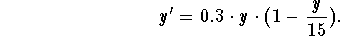Note that this equation is also autonomous!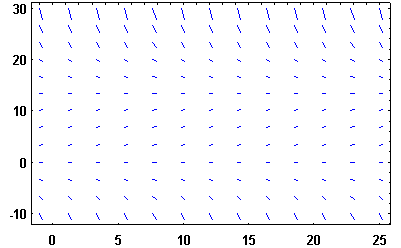The solutions of this logistic equation have the following form: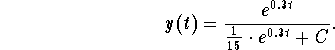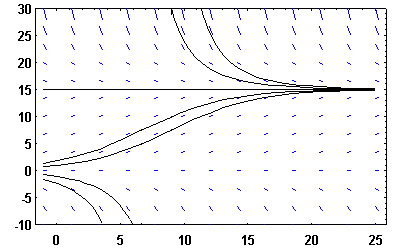As a last example, we consider the non-autonomous differential equation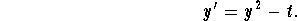Now the slope field looks slightly more complicated.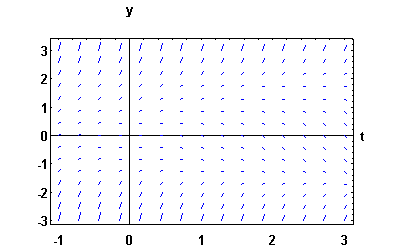Here is the same slope field again. What is special about the points on the red parabola?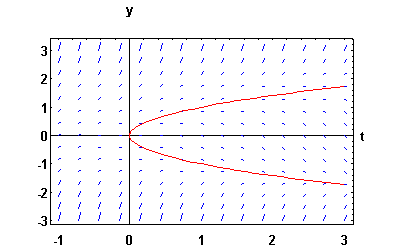### If you would like more practice, click on Example.[Differential Equations] [Linear Equations] [Separable Equations]
[Geometry] [Algebra] [Trigonometry ]
[Calculus] [Complex Variables] [Matrix Algebra]S.O.S MATHematics home page

Do you need more help? Please post your question on our S.O.S. Mathematics CyberBoard.Author: Helmut Knaust